# Propositional Logic 1 Propositional logic Logical constants true

• Slides: 13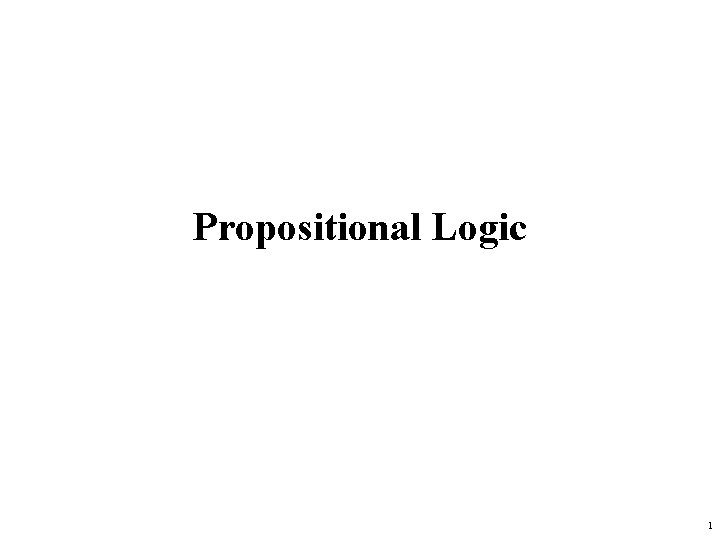Propositional Logic 1Propositional logic • • Logical constants: true, false Propositional symbols: P, Q, S, . . . (atomic sentences) Wrapping parentheses: ( … ) Sentences are combined by connectives: . . . and . . . or . . . implies . . is equivalent . . . not [conjunction] [disjunction] [implication / conditional] [biconditional] [negation] • Literal: atomic sentence or negated atomic sentence 2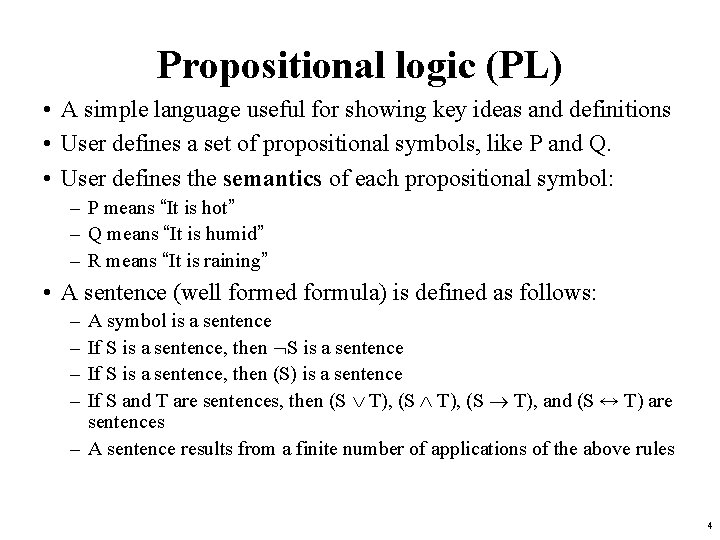Propositional logic (PL) • A simple language useful for showing key ideas and definitions • User defines a set of propositional symbols, like P and Q. • User defines the semantics of each propositional symbol: – P means “It is hot” – Q means “It is humid” – R means “It is raining” • A sentence (well formed formula) is defined as follows: – – A symbol is a sentence If S is a sentence, then S is a sentence If S is a sentence, then (S) is a sentence If S and T are sentences, then (S T), and (S ↔ T) are sentences – A sentence results from a finite number of applications of the above rules 4Some terms • The meaning or semantics of a sentence determines its interpretation. • Given the truth values of all symbols in a sentence, it can be “evaluated” to determine its truth value (True or False). • A model for a KB is a “possible world” (assignment of truth values to propositional symbols) in which each sentence in the KB is True. 6More terms • A valid sentence or tautology is a sentence that is True under all interpretations, no matter what the world is actually like or what the semantics is. Example: “It’s raining or it’s not raining. ” • An inconsistent sentence or contradiction is a sentence that is False under all interpretations. The world is never like what it describes, as in “It’s raining and it’s not raining. ” • P entails Q, written P |= Q, means that whenever P is True, so is Q. In other words, all models of P are also models of Q. 7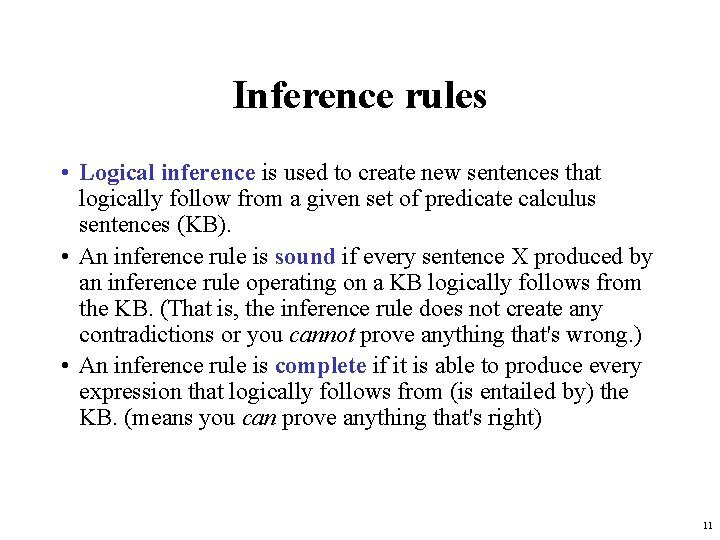Inference rules • Logical inference is used to create new sentences that logically follow from a given set of predicate calculus sentences (KB). • An inference rule is sound if every sentence X produced by an inference rule operating on a KB logically follows from the KB. (That is, the inference rule does not create any contradictions or you cannot prove anything that's wrong. ) • An inference rule is complete if it is able to produce every expression that logically follows from (is entailed by) the KB. (means you can prove anything that's right) 11Sound rules of inference • Here are some examples of sound rules of inference – A rule is sound if its conclusion is true whenever the premise is true • Each can be shown to be sound using a truth table RULE PREMISE CONCLUSION Modus Ponens And Introduction And Elimination Double Negation Unit Resolution A, A B A, B A B A A B, B C B A A A A C 12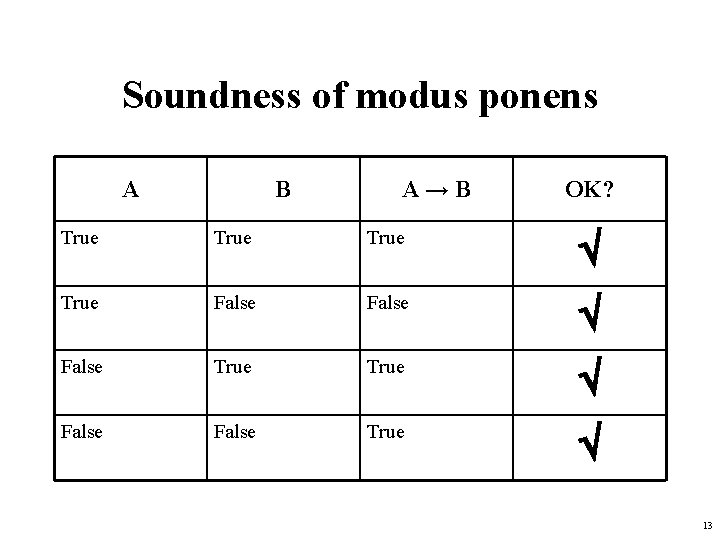Soundness of modus ponens A B A→B OK? True False True False True 13Soundness of the resolution inference rule From the rightmost three columns of this truth table, we can see that (α v β )ʌ ( ┐β v γ ) → α v γ is valid regardless of the truth values assigned to α, β or γ 14Proving things • A proof is a sequence of sentences, where each sentence is either a premise or a sentence derived from earlier sentences in the proof by one of the rules of inference. • The last sentence is theorem (also called goal or query) that we want to prove. • Example for the “weather problem” given above. 1 Hu Premise “It is humid” 2 Hu Ho Premise “If it is humid, it is hot” 3 Ho Modus Ponens(1, 2) “It is hot” 4 (Ho Hu) R Premise “If it’s hot & humid, it’s raining” 5 Ho Hu And Introduction(1, 3) “It is hot and humid” 6 R Modus Ponens(4, 5) “It is raining” 15Horn sentences • A Horn sentence or Horn clause has the form: P 1 P 2 P 3. . . Pn Q or alternatively (P Q) = ( P Q) P 1 P 2 P 3. . . Pn Q where Ps and Q are non-negated atoms • To get a proof for Horn sentences, apply Modus Ponens repeatedly until nothing can be done • We will use the Horn clause form later 16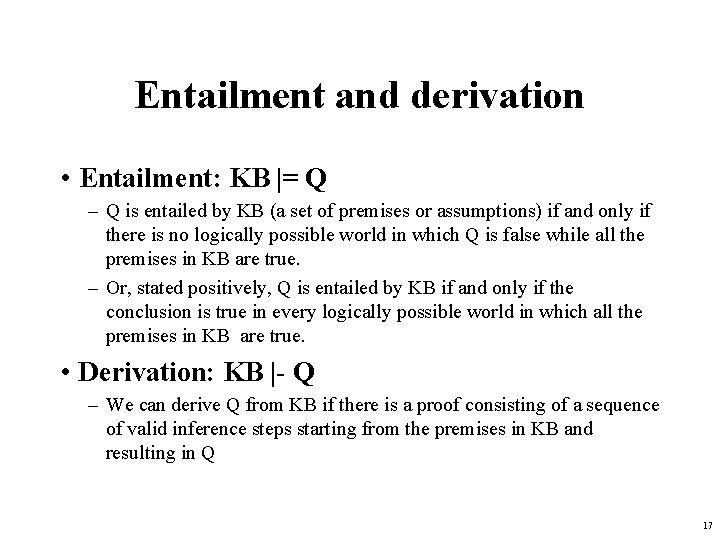Entailment and derivation • Entailment: KB |= Q – Q is entailed by KB (a set of premises or assumptions) if and only if there is no logically possible world in which Q is false while all the premises in KB are true. – Or, stated positively, Q is entailed by KB if and only if the conclusion is true in every logically possible world in which all the premises in KB are true. • Derivation: KB |- Q – We can derive Q from KB if there is a proof consisting of a sequence of valid inference steps starting from the premises in KB and resulting in Q 17Two important properties for inference Soundness: If KB |- Q then KB |= Q – If Q is derived from a set of sentences KB using a given set of rules of inference, then Q is entailed by KB. – Hence, inference produces only real entailments, or any sentence that follows deductively from the premises is valid. Completeness: If KB |= Q then KB |- Q – If Q is entailed by a set of sentences KB, then Q can be derived from KB using the rules of inference. – Hence, inference produces all entailments, or all valid sentences can be proved from the premises. 18